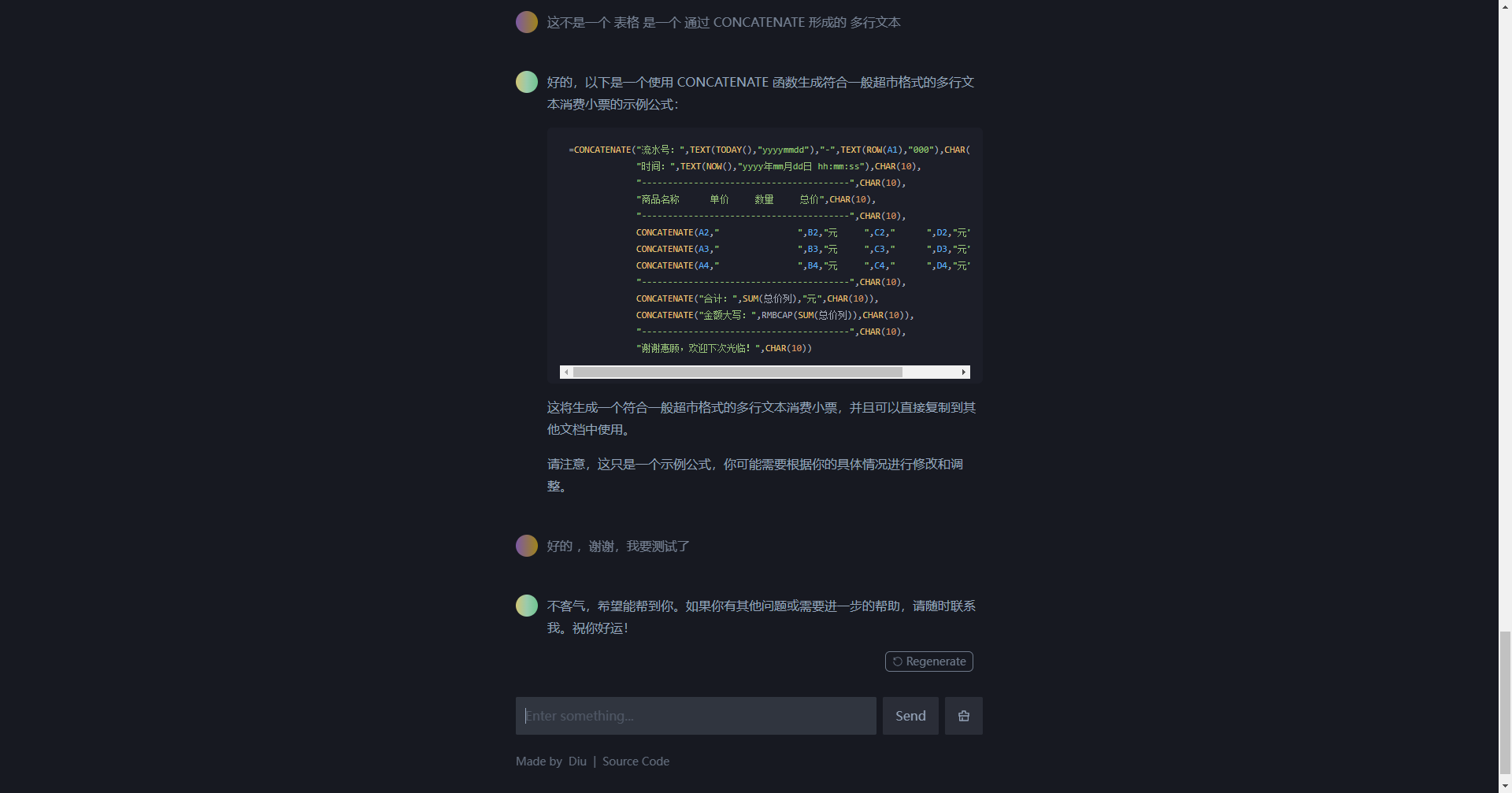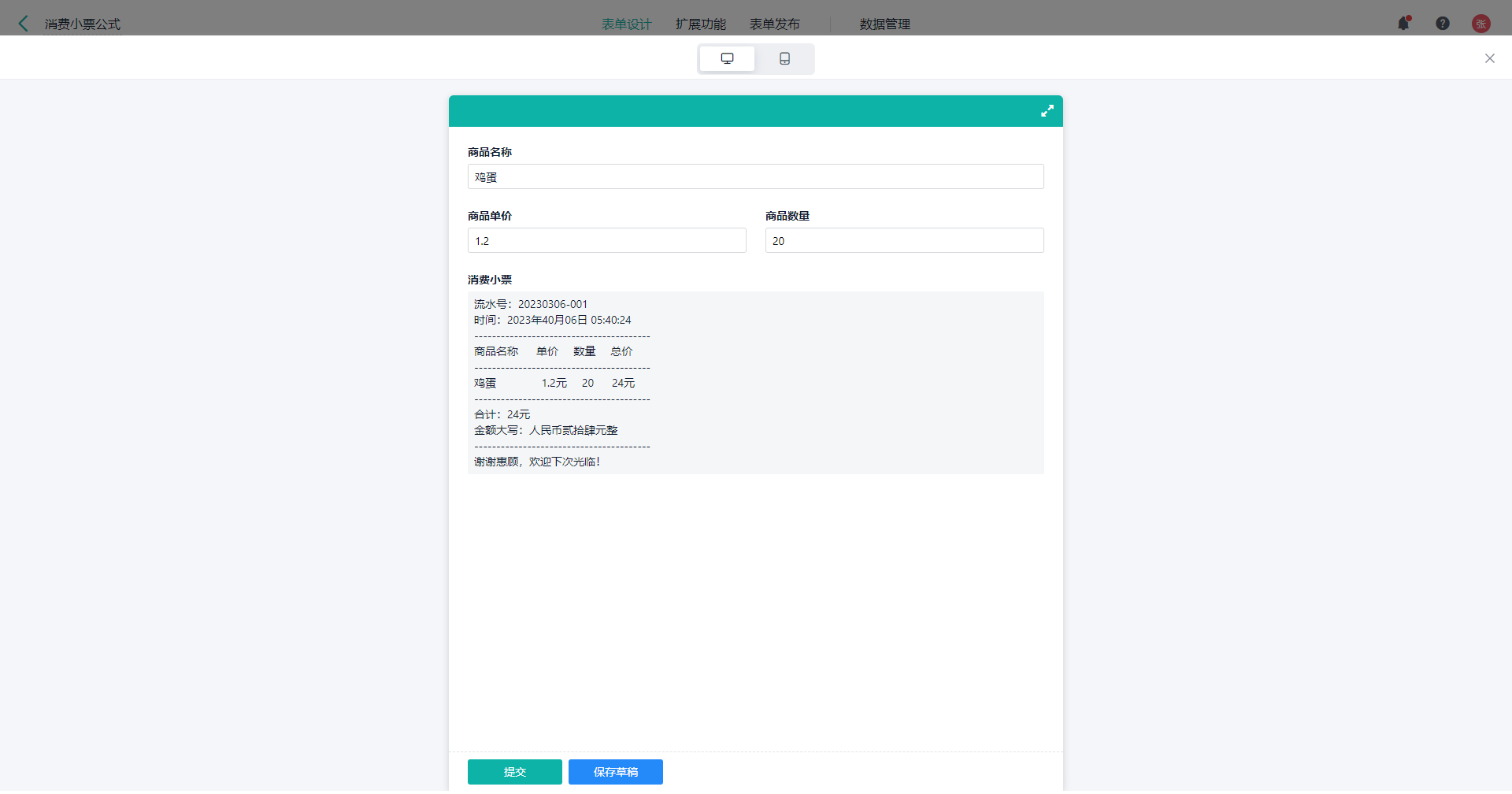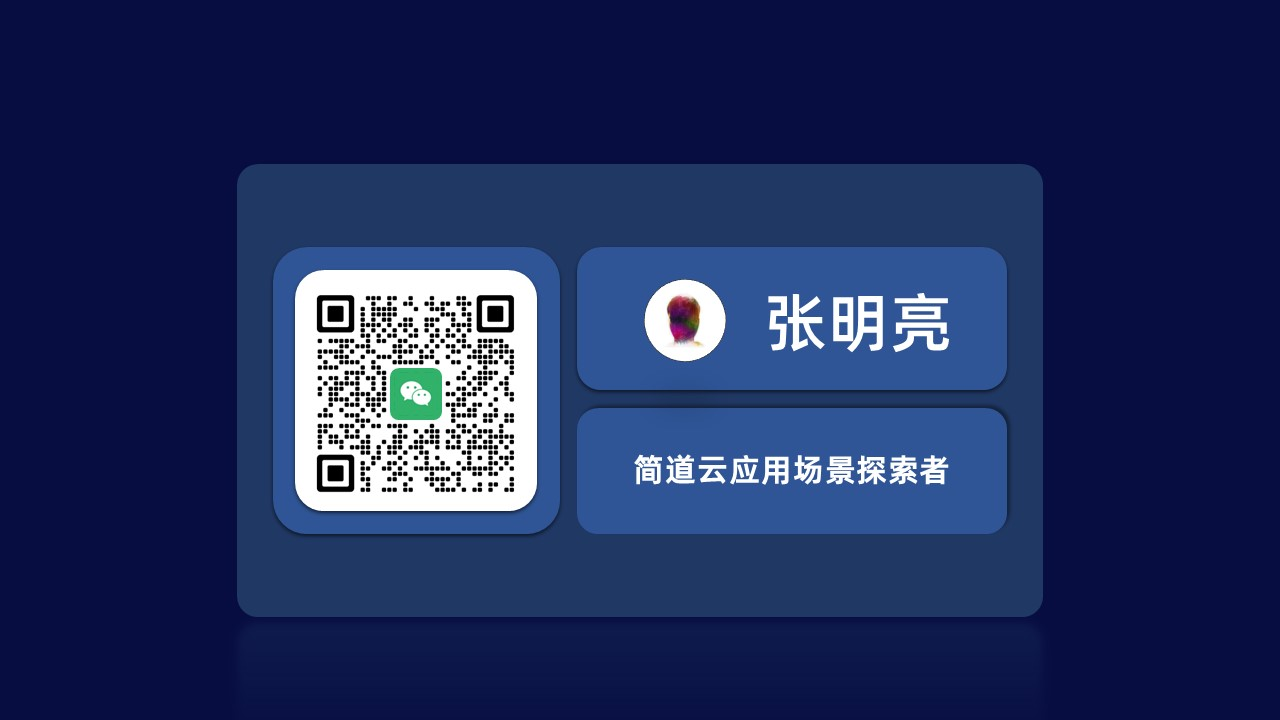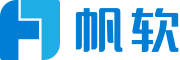# 分享：让ChatGPT帮你写公式 这事儿够不够颠覆

## 实现效果：## 协同过程：

ChatGPT Demo

Based on OpenAI API (gpt-3.5-turbo).

`=数量*单价`

`=SUM(总价列)`

`=SUMIF(日期列,日期,总价列)`

`=SUM(总价列)`

1. 首先，在表格中添加以下列：流水号、商品、数量、单价、总价。
2. 在流水号列中，使用以下公式生成流水号：

`=TEXT(TODAY(),"yyyymmdd")&"-"&TEXT(ROW(A1),"000")`

`=数量*单价`

1. 在表格的底部，使用以下公式计算所有商品的总价：

`=SUM(总价列)`

1. 在表格的底部，使用以下公式将总价转换为大写金额：

`=RMBCAP(SUM(总价列))`

`=TEXT(TODAY(),"yyyy年mm月dd日")`

1. 首先，在表格中添加以下列：流水号、商品、数量、单价、总价。
2. 在流水号列中，使用以下公式生成流水号：

`=CONCATENATE(TEXT(TODAY(),"yyyymmdd"),"-",TEXT(ROW(A1),"000"))`

`=CONCATENATE(数量," x ",单价," = ",数量*单价)`

`=CONCATENATE("总价：",SUM(总价列),"元（大写）：",RMBCAP(SUM(总价列)))`

`=CONCATENATE("日期：",TEXT(TODAY(),"yyyy年mm月dd日"))`

1. 首先，在表格中添加以下列：流水号、商品、数量、单价、总价。
2. 在流水号列中，使用以下公式生成流水号：

`=CONCATENATE("流水号：",TEXT(TODAY(),"yyyymmdd"),"-",TEXT(ROW(A1),"000"))`

`=CONCATENATE(A2,"（",B2,"）")`

`=数量*单价`

1. 在表格的底部，使用以下公式拼接所有商品的总价：

`=CONCATENATE("合计：",SUM(总价列),"元")`

`=CONCATENATE("金额大写：",RMBCAP(SUM(总价列)))`

`=CONCATENATE("时间：",TEXT(NOW(),"yyyy年mm月dd日 hh:mm:ss"))`

``````=CONCATENATE("流水号：",TEXT(TODAY(),"yyyymmdd"),"-",TEXT(ROW(A1),"000"),CHAR(10),
"时间：",TEXT(NOW(),"yyyy年mm月dd日 hh:mm:ss"),CHAR(10),
"----------------------------------------",CHAR(10),
"商品名称      单价     数量     总价",CHAR(10),
"----------------------------------------",CHAR(10),
CONCATENATE(A2,"               ",B2,"元     ",C2,"      ",D2,"元"),CHAR(10),
CONCATENATE(A3,"               ",B3,"元     ",C3,"      ",D3,"元"),CHAR(10),
CONCATENATE(A4,"               ",B4,"元     ",C4,"      ",D4,"元"),CHAR(10),
"----------------------------------------",CHAR(10),
CONCATENATE("合计：",SUM(总价列),"元",CHAR(10)),
CONCATENATE("金额大写：",RMBCAP(SUM(总价列)),CHAR(10)),
"----------------------------------------",CHAR(10),
"谢谢惠顾，欢迎下次光临！",CHAR(10))
``````

## 原始公式：

``````=CONCATENATE("流水号：",TEXT(TODAY(),"yyyymmdd"),"-",TEXT(ROW(A1),"000"),CHAR(10),
"时间：",TEXT(NOW(),"yyyy年mm月dd日 hh:mm:ss"),CHAR(10),
"----------------------------------------",CHAR(10),
"商品名称      单价     数量     总价",CHAR(10),
"----------------------------------------",CHAR(10),
CONCATENATE(A2,"               ",B2,"元     ",C2,"      ",D2,"元"),CHAR(10),
CONCATENATE(A3,"               ",B3,"元     ",C3,"      ",D3,"元"),CHAR(10),
CONCATENATE(A4,"               ",B4,"元     ",C4,"      ",D4,"元"),CHAR(10),
"----------------------------------------",CHAR(10),
CONCATENATE("合计：",SUM(总价列),"元",CHAR(10)),
CONCATENATE("金额大写：",RMBCAP(SUM(总价列)),CHAR(10)),
"----------------------------------------",CHAR(10),
"谢谢惠顾，欢迎下次光临！",CHAR(10))``````

## 更多内容：

#### 汇总：论坛中发表过的所有帖子分享一下怎么访问chatgpt
 本版积分规则 回帖后跳转到最后一页隐私政策